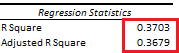# Linear Regression: Coefficient of Determination

Last Update: February 21, 2022

Coefficient of determination or r-squared  is used to evaluate linear regression goodness of fit by estimating the percentage of the variance from dependent variable  explained by its relationship with independent variable . When linear regression has two or more independent variables , it is known as coefficient of multiple determination or multiple r-squared.

As example, we can fit a three-variable multiple linear regression with formula . Then, we can estimate its coefficient of multiple determination with formula . Residuals sum of squares  with formula is estimated as the sum of squared regression residuals . Regression residuals  with formula are estimated as the differences between actual  and fitted  values. Total sum of squares  with formula is calculated as the sum of squared differences between dependent variable  values and their arithmetic mean .

Adding independent variables  is likely to increase coefficient of multiple determination. Therefore, we can estimate adjusted coefficient of multiple determination or adjusted multiple r-squared with formula  by taking model degrees of freedom reduction into consideration. Total degrees of freedom  with formula  is the number of observations  minus the constant term. Residuals degrees of freedom  with formula  is the number of observations  minus number of independent variables  minus the constant term.

Below, we find an example of estimated coefficients of multiple determination from multiple linear regression of house price explained by its lot size and number of bedrooms .Table 1. Microsoft Excel® estimated coefficients of multiple determination from multiple linear regression of house price explained by its lot size and number of bedrooms.

Courses

My online courses are hosted at Teachable website.

For more details on this concept, you can view my Linear Regression Courses.

 Data Description: Sales prices of houses sold in the city of Windsor, Canada, during July, August and September, 1987.

Original Source: Anglin, P., and Gencay, R. (1996). Semiparametric Estimation of a Hedonic Price Function. Journal of Applied Econometrics, 11, 633–648.

Source: AER R Package HousePrices Object. Christian Kleiber and Achim Zeileis. (2008). Applied Econometrics with R. Springer-Verlag, New York.

+